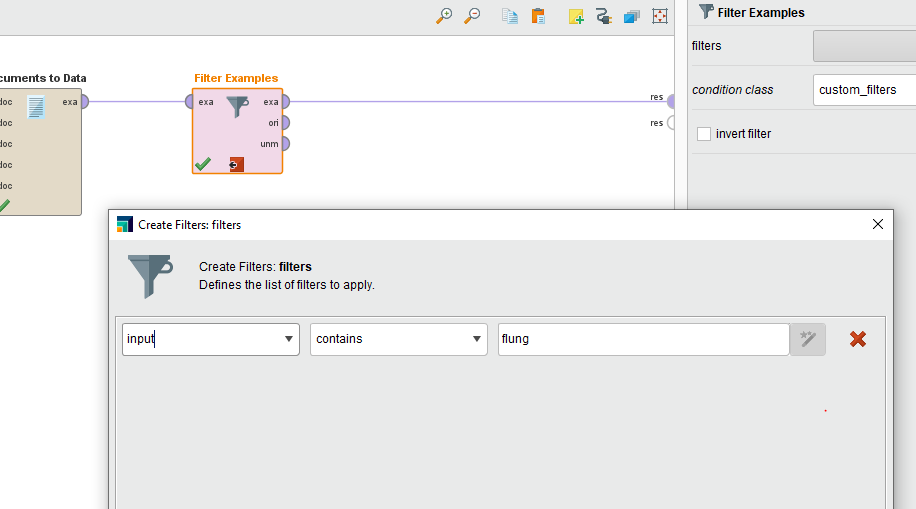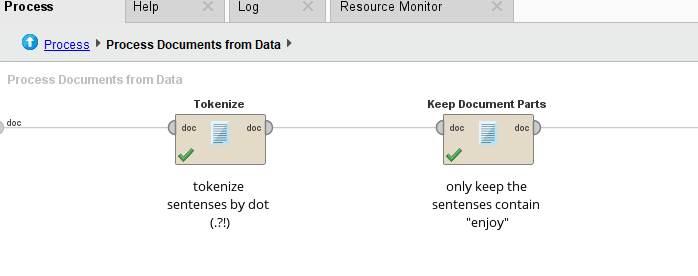# How to extract/filter text elements using regex?

Member Posts: 2Newbie
Dear community,

Currently I've been trying to use regular expressions in my RAPIDMINER model to filter extracts from a text. The text is extracted from excel under one content attribute. Each text is extracted from one of these cells in excel. I would like to extract specific sentences using regular expressions ([^.?!]*(?<=[.?\s!])flung(?=[\s.?!])[^.?!]*[.?!]) from these text, based on one word that must be included. I've tried to use 'select attributes' and 'filter examples', but I don't get the right output (i.e., only those sentences that contain one of the words). I would also need to correct for multiple words within one sentence (perhaps using the use exception?). Perhaps you have an idea how to integrate such process into the model? Any help is greatly appreciated!
Tagged:

• Administrator, Employee, RapidMiner Certified Analyst, RapidMiner Certified Expert, Member Posts: 363RM Data Scientist
You can "replace tokens" when you process text documents.
Also "filter example" could also work like thisIf you need to detect the keywords and find the location of the keywords, NLP Tagger from this extension would be useful.

HTH!
• Member Posts: 2Newbie
Dear yyhuang, thank you so much for your quick reply and the provided solution. The solution you provided does allow me to consider only those texts that contain specific words. I do, however, would like to extract only those sentences out of the text that contain such specific word, not the whole text. Is there any way to filter within such text as well? Thank you in advance!
• Administrator, Employee, RapidMiner Certified Analyst, RapidMiner Certified Expert, Member Posts: 363RM Data Scientist
Thank you @rmwolter for the feedback. Yes, the sentences need to be split. If we "tokenize" by . ! ? and apply the regex to detect the keywords of interest.Another option is to use NLP Tagger to find all keywords positions and then apply filter on NLP Tagger results.

HTH!
• Administrator, Employee, RapidMiner Certified Analyst, Member Posts: 271Unicorn
Hi @rmwolter

You could tokenize your text by linguistic sentences and then apply the filter tokens by content operator.

Hope this example helps you.

```<?xml version="1.0" encoding="UTF-8"?><process version="9.10.010">
<context>
<input/>
<output/>
<macros/>
</context>
<operator activated="true" class="process" compatibility="9.10.010" expanded="true" name="Process">
<parameter key="logverbosity" value="init"/>
<parameter key="random_seed" value="-1"/>
<parameter key="send_mail" value="never"/>
<parameter key="process_duration_for_mail" value="30"/>
<parameter key="encoding" value="UTF-8"/>
<process expanded="true">
<operator activated="true" class="retrieve" compatibility="9.10.010" expanded="true" height="68" name="Retrieve 01 - Coded Sentiments" width="90" x="112" y="34">
<parameter key="repository_entry" value="//Community Samples/Community Webinar Materials/Webinar on Hotel Sentiment Analysis/data/01 - Coded Sentiments"/>
</operator>
<operator activated="true" class="select_attributes" compatibility="9.10.010" expanded="true" height="82" name="Select Attributes" width="90" x="246" y="34">
<parameter key="attribute_filter_type" value="subset"/>
<parameter key="attribute" value=""/>
<parameter key="attributes" value="Text"/>
<parameter key="use_except_expression" value="false"/>
<parameter key="value_type" value="attribute_value"/>
<parameter key="use_value_type_exception" value="false"/>
<parameter key="except_value_type" value="time"/>
<parameter key="block_type" value="attribute_block"/>
<parameter key="use_block_type_exception" value="false"/>
<parameter key="except_block_type" value="value_matrix_row_start"/>
<parameter key="invert_selection" value="false"/>
<parameter key="include_special_attributes" value="true"/>
</operator>
<operator activated="true" class="nominal_to_text" compatibility="9.10.010" expanded="true" height="82" name="Nominal to Text" width="90" x="380" y="34">
<parameter key="attribute_filter_type" value="all"/>
<parameter key="attribute" value=""/>
<parameter key="attributes" value=""/>
<parameter key="use_except_expression" value="false"/>
<parameter key="value_type" value="nominal"/>
<parameter key="use_value_type_exception" value="false"/>
<parameter key="except_value_type" value="file_path"/>
<parameter key="block_type" value="single_value"/>
<parameter key="use_block_type_exception" value="false"/>
<parameter key="except_block_type" value="single_value"/>
<parameter key="invert_selection" value="false"/>
<parameter key="include_special_attributes" value="false"/>
</operator>
<operator activated="true" class="text:process_document_from_data" compatibility="9.4.000" expanded="true" height="82" name="Process Documents from Data" width="90" x="514" y="34">
<parameter key="create_word_vector" value="false"/>
<parameter key="vector_creation" value="TF-IDF"/>
<parameter key="keep_text" value="true"/>
<parameter key="prune_method" value="none"/>
<parameter key="prune_below_percent" value="3.0"/>
<parameter key="prune_above_percent" value="30.0"/>
<parameter key="prune_below_rank" value="0.05"/>
<parameter key="prune_above_rank" value="0.95"/>
<parameter key="datamanagement" value="double_sparse_array"/>
<parameter key="data_management" value="auto"/>
<parameter key="select_attributes_and_weights" value="false"/>
<list key="specify_weights"/>
<process expanded="true">
<operator activated="true" class="text:tokenize" compatibility="9.4.000" expanded="true" height="68" name="Tokenize" width="90" x="179" y="34">
<parameter key="mode" value="linguistic sentences"/>
<parameter key="characters" value=".:"/>
<parameter key="language" value="English"/>
<parameter key="max_token_length" value="3"/>
</operator>
<operator activated="true" class="text:filter_tokens_by_content" compatibility="9.4.000" expanded="true" height="68" name="Filter Tokens (by Content)" width="90" x="313" y="34">
<parameter key="condition" value="contains"/>
<parameter key="string" value="time"/>
<parameter key="case_sensitive" value="true"/>
<parameter key="invert condition" value="false"/>
</operator>
<connect from_port="document" to_op="Tokenize" to_port="document"/>
<connect from_op="Tokenize" from_port="document" to_op="Filter Tokens (by Content)" to_port="document"/>
<connect from_op="Filter Tokens (by Content)" from_port="document" to_port="document 1"/>
<portSpacing port="source_document" spacing="0"/>
<portSpacing port="sink_document 1" spacing="0"/>
<portSpacing port="sink_document 2" spacing="0"/>
</process>
</operator>
<connect from_op="Retrieve 01 - Coded Sentiments" from_port="output" to_op="Select Attributes" to_port="example set input"/>
<connect from_op="Select Attributes" from_port="example set output" to_op="Nominal to Text" to_port="example set input"/>
<connect from_op="Nominal to Text" from_port="example set output" to_op="Process Documents from Data" to_port="example set"/>
<connect from_op="Process Documents from Data" from_port="example set" to_port="result 1"/>
<portSpacing port="source_input 1" spacing="0"/>
<portSpacing port="sink_result 1" spacing="0"/>
<portSpacing port="sink_result 2" spacing="0"/>
</process>
</operator>
</process>```